How to play NC-Backgammon

We use the classical backgammon board with 30 checkers as it is. The rules of the moving of the checkers are also same as the classical one. We can say that the NC-Backgammon is a new variant of an existing classical backgammon. The only exception is the lack of the rolling of dices. Other than that, all the rules of the NC-Backgammon are the same as classical backgammon. Therefore, we shall assume the reader already knows the existing classical backgammon, and we are not going to give here the rules of how to play the backgammon.

In order to play the game, we need a pair of numbers. Normally, in the classical backgammon, the dices are used to obtain the number pairs. To do this the six numbers are written on the bar of the table of the classical backgammon, the Red player has a red arrow and the player Blue player has a blue arrow to indicator own number on the numbered bar as in the following figure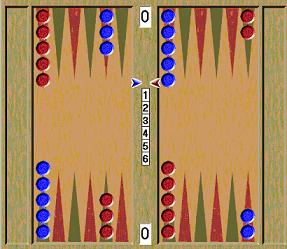The board is the opening positions of the NC-Backgammon.

The rules

1. The players are ordered by a way, such as drawing of lots, to know who the first player is.
2. Each player choose a different numbers respectively and put own indicator into numbered bar to indicate own chose number to form the starting number pair. The player has not moved own checkers yet with this starting number pair.
3. Then, in their turn, each player has to remove own indicator to indicate own chose number such that the number can not be indicated by opponent indicator, it may be done only two times during the game to get the doubling, if necessary, and then get their number pair which showed by both indicators. The player plays with this number pair as if the pair is obtained by rolling two dice.
It is clear to see that, in any turn, when a player chooses a number from the alternative set under the conditions with a good strategy, the player has to consider previous pair, next pair, both players possible moving, and both players' checkers positions on a board.

Example

Let us say that the Red player has won the toss and he gets to choose number 2 from the six numbers. Then Blue chooses number 5 from the remaining ones. Therefore, the numbers (2,5) is the starting number piar as follows;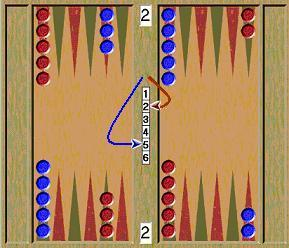Red Turn: Red chooses another number 3 from the remaining ones and changes this with number 2 in the starting number piar, then Red get own number pair (3,5) and moves own checkers according to this number pair as follows;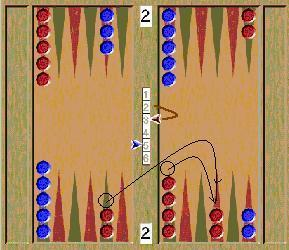Blue Turn: Blue chooses number 6 which is different than numbers of final number pair (3,5), that is, it is not indicated both indicators. Blue changes this with number 5 in the final number piar (Red's number pair), then Blue get own number pair (3,6) and moves own checkers according to this number pair as follows;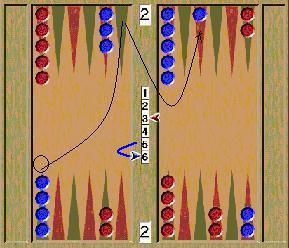Red Turn: Red chooses number 6 which is same one of the final number pair (3,6), each player can do this only two times during the game to get the doubling, then Red get own number pair (6,6) and moves own checkers according to this number pair as follows;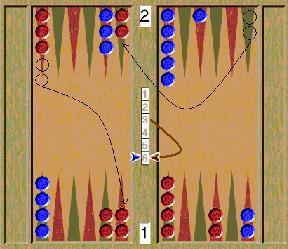Blue Turn: Blue chooses number 3, then by the same way Blue get own number pair (6,3) and play with this number pair as follows;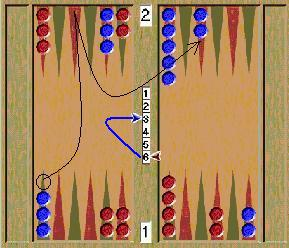Red Turn: Red chooses 1, and then get own number pair (1,3) and play with this as follows;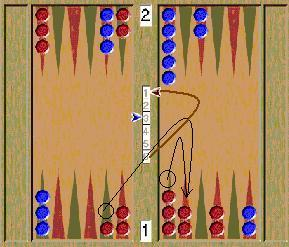Blue Turn: Blue chooses 6, and play with (1,6) as follows;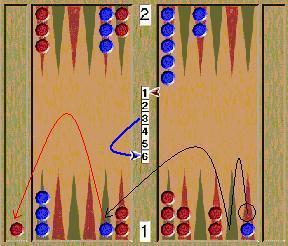Red Turn: Red chooses 5, and play with (5,6) as follows;Blue Turn: Blue chooses 3, and play with (5,3) as follows;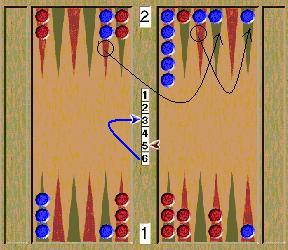Red Turn: Red chooses 2, and play with (2,3) as follows;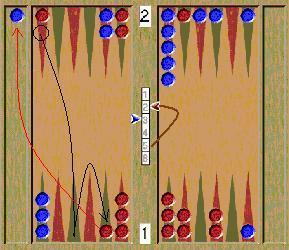Blue Turn: Blue chooses 1, and play with (2,1) as follows;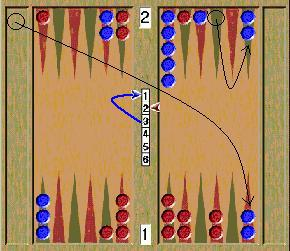Red Turn: Red chooses 6, and play with (6,1) as follows;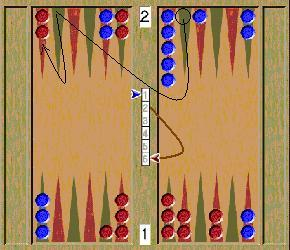Blue Turn: Blue chooses 5, and play with (6,5) as follows;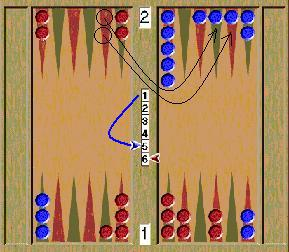Red Turn: Red chooses 4, and play with (4,5) as follows;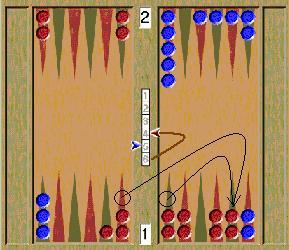Blue Turn: Blue chooses 6, and play with (4,6) as follows;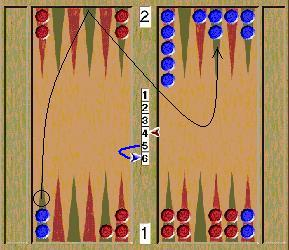Red Turn: Red chooses 5, and play with (5,6) as follows;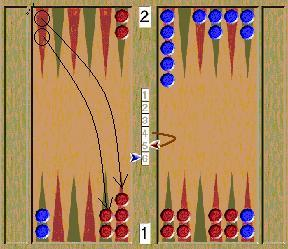and so on. This procedure is continuing until game is finished.